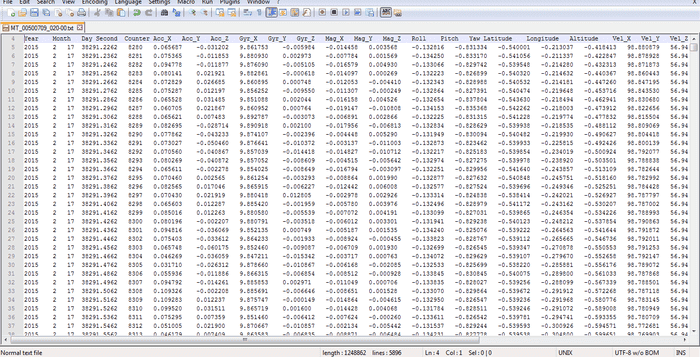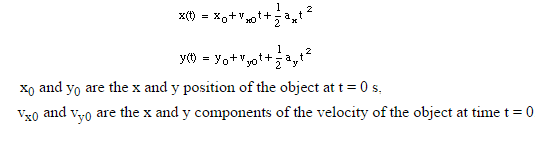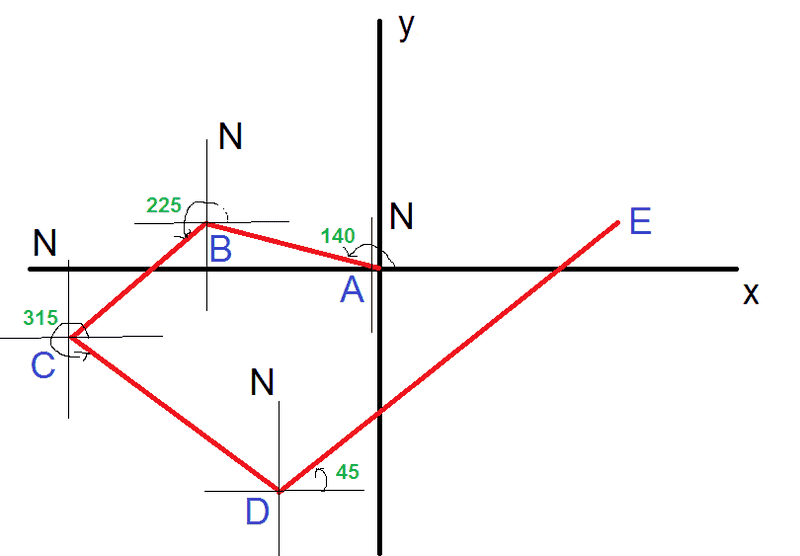# Motion in plane

## Homework Statement

I'm doing project using xsens MTi-G.
Data file example:I must plot motion in plane (decart coordinate system), without z component.
Measurment is taken after 0.01 s. To do the task, I should use acceleration and velosity data.

## Homework Equations## The Attempt at a Solution

Can I use those equations? I guess they are for constant acceleration. Or am I wrong?

DEvens
Gold Member
Those equations are for constant acceleration. And looking at the data file you posted part of, it looks like your acceleration is not constant.

Depending on your level of calculus, you have a few ways of proceeding. If your calculus is introductory you could approximate that the acceleration is constant over each measurement interval. Then you can use those equations over each interval. So at time=t1, say the first row in your file, you could have x1, Vx1, and ax1. Then evolve the position forward in time by that one interval using those equations. That will get you from x(t1) to x(t2).

This is essentially Euler's method.
http://en.wikipedia.org/wiki/Euler_method

So there are some tricky bits to that. The time you need to use will be the time after time t1. So t1 looks to be 38291.2262 and t2 looks to be 38291.2362. So the t in the equation is the amount of time from t1 to t2, or 0.01 seconds. So you would apply the initial velocity and acceleration for that long.

x(t1) is presumably known.
x(t2) = x(t1) + Vx(t1) * 0.01 + 1/2 ax(t1) * (0.01)^2

And Vx(t1) and ax(t1) are the values from your data. Then you get x(t3) by evolving forward from t2 to t3 using he next time interval. You might do this in a spreadsheet such as MS Excel. Or maybe your "xsens MTi-G" will do it for you.

If your calculus is a bit more advanced then you could deal with the equations for non-constant acceleration. Velocity is the integral of acceleration, and position is the integral of velocity. And you could do some kind of curve-fitting to get the acceleration as a function of time between measurement points. This will presumably be more accurate than the previous method. Possibly your "xsens MTi-G" will do that for you as well.

Ok, it's clear about that. But now I'm trying to do more advanced calculations. I want to include heading and distance in my calculations. Let's say I integrate resulting acceleration vector twice to get distace.
resulting_acc = sqrt(accX^2 + acc_Y^2)
To get heading, I use magnetometer data, so direction = atan2(Mag_Y,Mag_X), then I convert it to 0-360 degrees.
Where exactly this heading angle is on the plane?
Is this angle to north like in the picture?